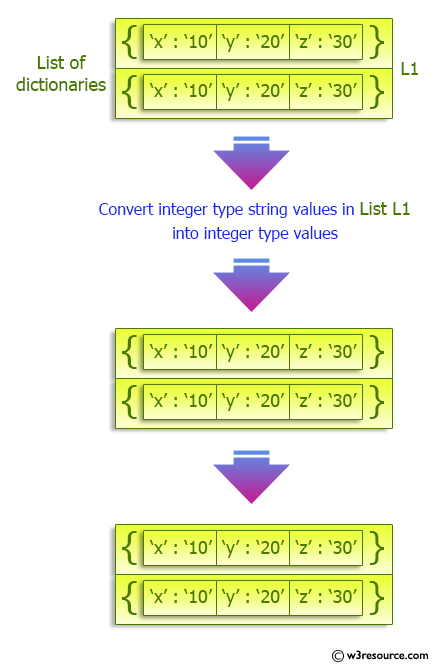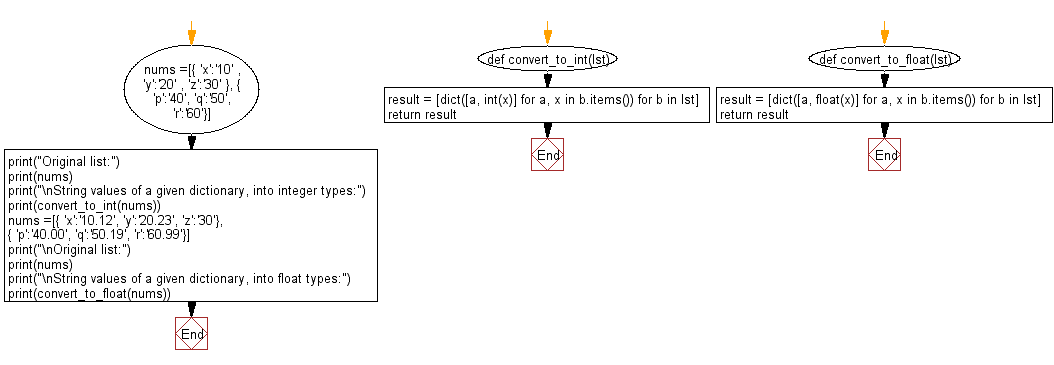﻿ Python: Convert string values of a given dictionary, into integer/float datatypes - w3resource# Python: Convert string values of a given dictionary, into integer/float datatypes

## Python dictionary: Exercise-49 with Solution

Write a Python program to convert string values of a given dictionary, into integer/float datatypes.

Sample Solution:

Python Code:

``````def convert_to_int(lst):
result = [dict([a, int(x)] for a, x in b.items()) for b in lst]
return result

def convert_to_float(lst):
result = [dict([a, float(x)] for a, x in b.items()) for b in lst]
return result

nums =[{ 'x':'10' , 'y':'20' , 'z':'30' }, { 'p':'40', 'q':'50', 'r':'60'}]
print("Original list:")
print(nums)
print("\nString values of a given dictionary, into integer types:")
print(convert_to_int(nums))
nums =[{ 'x':'10.12', 'y':'20.23', 'z':'30'}, { 'p':'40.00', 'q':'50.19', 'r':'60.99'}]
print("\nOriginal list:")
print(nums)
print("\nString values of a given dictionary, into float types:")
print(convert_to_float(nums))
```
```

Sample Output:

```Original list:
[{'x': '10', 'y': '20', 'z': '30'}, {'p': '40', 'q': '50', 'r': '60'}]

String values of a given dictionary, into integer types:
[{'x': 10, 'y': 20, 'z': 30}, {'p': 40, 'q': 50, 'r': 60}]

Original list:
[{'x': '10.12', 'y': '20.23', 'z': '30'}, {'p': '40.00', 'q': '50.19', 'r': '60.99'}]

String values of a given dictionary, into float types:
[{'x': 10.12, 'y': 20.23, 'z': 30.0}, {'p': 40.0, 'q': 50.19, 'r': 60.99}]
```

Pictorial Presentation:Flowchart:## Visualize Python code execution:

The following tool visualize what the computer is doing step-by-step as it executes the said program:

Python Code Editor:

Have another way to solve this solution? Contribute your code (and comments) through Disqus.

What is the difficulty level of this exercise?

Test your Programming skills with w3resource's quiz.

﻿

## Python: Tips of the Day

Invokes the provided function after ms milliseconds:

Example:

```from time import sleep

def tips_delay(fn, ms, *args):
sleep(ms / 1000)
return fn(*args)
print(tips_delay(
lambda x: print(x),
1000,
'w3r'
))
```

Output:

```w3r
None
```